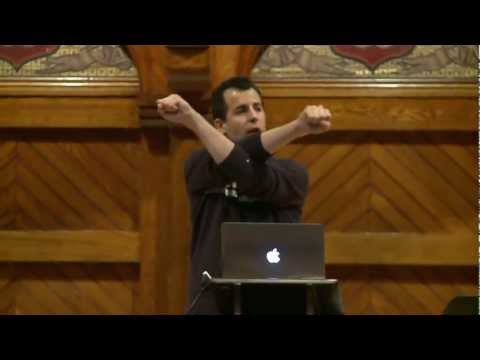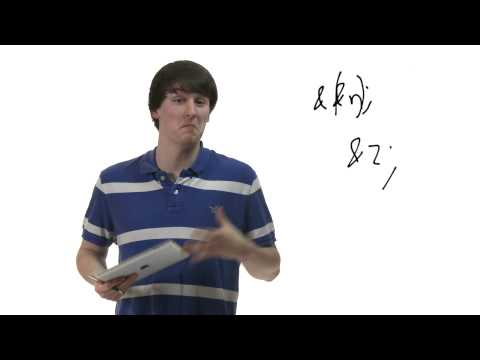# Pointers

## Notes

The C programming language gives us the ability to directly manipulate the contents of memory addresses via pointers. Unfortunately, this power brings with it the freedom to screw things up spectacularly as simple errors can corrupt the state of your program in ways that make debugging difficult. The goal of these slides is to make sure that you understand exactly what you're doing when you use pointers in your code.

The iconic clay figure depicted in this slide is Binky of the claymation Pointer Fun with Binky.

Code and data for your program are stored in random-access memory (RAM), which is basically a huge array of 1 byte (8 bits) blocks. Each block is associated with a hexadecimal number that represents its memory address.

Just as ints are variables that store integers and floats are variables that store floating-point values, a pointer is a variable that stores memory addresses. In the appliance (a 32-bit operating), a memory address is 4 bytes, so it makes sense that a pointer is also 4 bytes.

You should now be able to understand this comic!

Here's how to declare a pointer in C.

Remember that a pointer's value is a memory address. Its type then describes the data located at that address.

So in the first example, int* x declares a pointer to 4 bytes of memory that will contain an integer.

& is the reference, or address-of, operator. It returns the address in memory at which a variable is stored.

* is the dereference operator. A pointer's value is a memory address. When the dereference operator is applied to a pointer, it returns the data stored at that memory address.

Here are examples of the referencing and dereferencing operators in use. Let's think carefully about what's going on under the hood as each line executes.

• The first line declares an integer called x. 4 bytes are allotted for x at memory address 0x04, and the value 5 is stored there.
• The second line declares an int pointer called ptr. 4 bytes are allotted for ptr at memory address 0x08, and the address of x is stored there.
• The third line declares an integer called copy. 4 bytes are allotted for copy at memory address 0x0C, and the value of the integer that ptr is pointing to is stored there.

Let's track the values of these variables as each line executes:

• In the first line, x is initialized to 5.
• In the second line, ptr is assigned x's memory address. x remains 5.
• In the third line, we dereference ptr (aka, go to the value it is pointing to) and change that value to 35.

Test yourself by tracking the values of these variables just as we did in the previous slide.

Adding or subtracting n adjusts the pointer by n * sizeof(<pointer type>) bytes. For example, in the code on this slide, y is a pointer to an int called x, which is stored at 0x04. Therefore, adding 1 to y shifts the value of y by 1 * sizeof(int) or 4 bytes.

Pay close attention to the syntax of this for loop. ptr starts out pointing to the first character of the string. Incrementing ptr shifts the pointer by 1 * sizeof(char)or 1 byte, moving ptr to the next character in the string.

After the loop executes, the length of the string will be printed.

Under the hood, an array is treated like a pointer to its first element, so standard pointer arithmetic applies:

*array is equivalent to array

*(array+1) is equivalent to array

*(array + 2) is equivalent to array.## Slides ( / )## Swap

Prerequisites:

Implement a swap function with prototype

``void swap(int* a, int* b)``

that swaps the values using pointers.

``````jharvard@run.cs50.net (~): ./a.out
x is 1
y is 2
Swapping...
Swapped!
x is 2
y is 1``````

Try out some pseudocode here!
```#include <stdio.h>

void swap(int* a, int* b)
{
// TODO
}

int main(void)
{
int x = 1;
int y = 2;
printf("x is %i\n", x);
printf("y is %i\n", y);
printf("Swapping...\n");
swap(&x, &y);
printf("Swapped!\n");
printf("x is %i\n", x);
printf("y is %i\n", y);
}
```

## Ascii Puzzle

Prerequisites:

Pass the seven `char`s to `printf()` so that executing your code will print the word "POINTER". Hint: You only need each `char` once.

``````int s = 65;
int* t = &s;

char c = 'I';
char N = s + 'P' - 'A';
char B = *t - s + N + 2;
char E = &s - t + 78;
char J = *t + 19;
char I = N + 'A' - s - 1;
char O = 347/5;``````

``````jharvard@run.cs50.net (~): ./a.out
POINTER``````

Try out some pseudocode here!
```#include <stdio.h>

int main(void)
{
int s = 65;
int* t = &s;

// TODO: Print "POINTER" using these chars

char C = 'I';
char N = s + 'P' - 'A';
char B = *t - s + N + 2;
char E = &s - t + 78;
char J = *t + 19;
char I = N + 'A' - s - 1;
char O = 347/5;
}
```

## strchr

Prerequisites:

Implement `strchr()`, a standard function that returns a substring of a C string that starts with a given character. If the character is not in the string, it should return `NULL`.

``````jharvard@run.cs50.net (~): ./a.out
String: happy cat
Character: a
Looking for substring...
Substring: appy cat``````

Try out some pseudocode here!
```#include <stdio.h>
#include <cs50.h>

char* my_strchr(char* str, char c)
{
// TODO
}

int main(void)
{
printf("String: ");
char* str = GetString();
printf("Character: ");
char c = GetChar();
printf("Looking for substring...\n");
char* result = my_strchr(str, c);
if (result == NULL)
{
printf("Couldn't find %c.\n", c);
}
else
{
printf("Substring: %s \n", result);
}
}
```

## Videos#### Monday, Week 4

An introduction to pointers#### Rob's Pointers Short

Rob gives a general overview